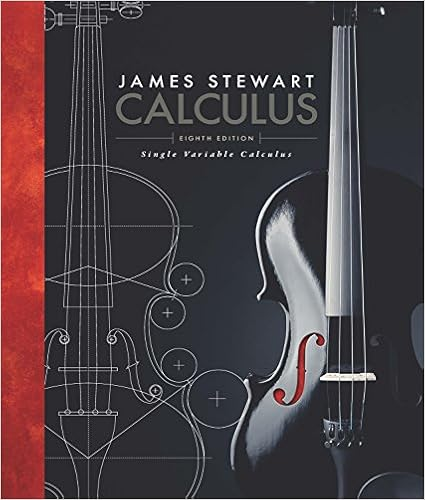# Lab Report 6 Solutions.pdf - MA103 Lab Report 6 Would you...

• Lab Report
• 2
• 100% (4) 4 out of 4 people found this document helpful

This preview shows page 1 - 2 out of 2 pages.

##### We have textbook solutions for you!
The document you are viewing contains questions related to this textbook.The document you are viewing contains questions related to this textbook.
Chapter 3 / Exercise 49
Single Variable Calculus
StewartExpert Verified
MA103 Lab Report 6 Would you like this assignment Graded? YES or NO Name: Student Number: Winter 2017 1. [13 marks ] Consider the function f ( x ) = (2 ° x ) e 2+ x : (a) State the domain of f: x 2 ( °1 ; 1 ) (b) Determine any x -intercepts and y -intercepts of f:
(c) Determine any horizontal asymptotes of f:
(d) Given that f 0 ( x ) = e x +2 (1 ° x ) ; determine the interval(s) on which f is increasing and on which f is decreasing and state any relative extrema :
(e) Given that f 00 ( x ) = ° xe x +2 ; determine the interval(s) on which f is concave up and on which f is concave down and state any points of in°ection.
(f) Using the information in parts (a)-(e), sketch the curve of f for x 2 [ ° 3 ;
##### We have textbook solutions for you!
The document you are viewing contains questions related to this textbook.The document you are viewing contains questions related to this textbook.
Chapter 3 / Exercise 49
Single Variable Calculus
StewartExpert Verified
•••